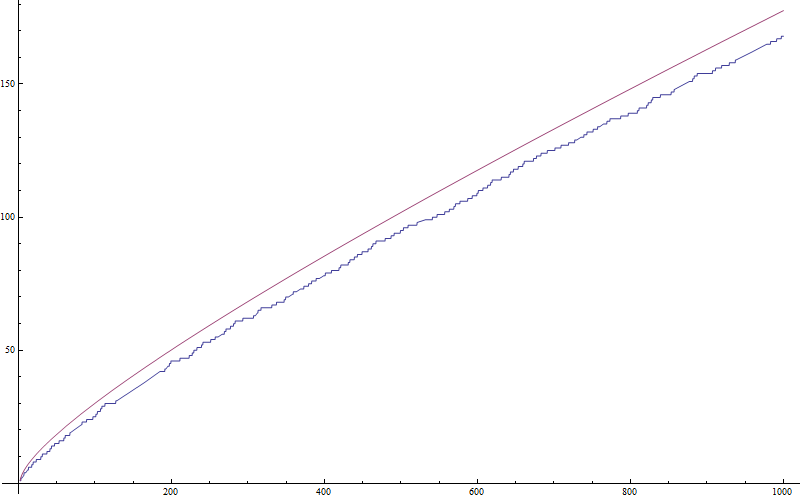## Pages

### Riemann and Large Numbers

Every now and then, I read in Ian Stewarts Hoard of Mathematical Treasures (to be more specific: in its German translation Professor Stewarts mathematische Schätze. Recently, I fell across Skewes' number.

From wikipedia:
In number theory, Skewes' number is any of several extremely large numbers used by the South African mathematician Stanley Skewes as upper bounds for the smallest natural number x for which
pi(x) > li(x),
where pi is the prime-counting function and li is the logarithmic integral function

(Note that the integrand has a singularity at t=1, and the integral must be evaluated as a Cauchy principal value).
It was already conjectured by Gauss that pi(x) behaves asymptotatically like li(x). This conjecture was provedy by Hadamard in 1896 (the same Hadamard who broought up the formulation of well-posedness of mathematical problems.

Anyway, for small numbers (small in a strange academic sense), pi(x) is smaller than li(x).li(x) (red), pi(x) (blue)
Littlewood proved in 1914 that there exists a number (albeit huge) for which pi(x) li(x), and Skewes showed in 1933 that there exists such a number with
x < 10^ (10^ (10^34)).
For this proof, Skewes needed the validity of Riemann's hypothesis (which is still not proved.)
Without the assumption of Riemann's hypothesis, Skewes succeeded to prove the existence of an x with
x< 10^ (10^ (10^963)) in 1955.

The estimates for smaller Skewes numbers have been reduced significantly. It seems that 10^316 is now a relevant order of magnitude.